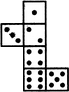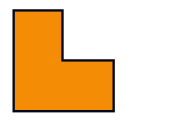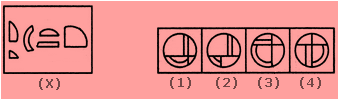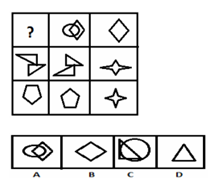# SilverZone - iRAO PDF Sample Papers for Class 7

Class 7 sample paper & practice questions for International Reasoning & Aptitude Olympiad (iRAO) level 1 are given below. Syllabus for level 1 is also mentioned for these exams. You can refer these sample paper & quiz for preparing for the exam.#### Resources:

##### Sample Questions from Olympiad Success:
 Q.1 Q.2 Q.3 Q.4 Q.5 Q.6 Q.7 Q.8 Q.9 Q.10
 Q.1 When the following figure is folded to form a cube, how many dots lie opposite the face bearing five dots?a) 1 b) 2 c) 3 d) 4
 Q.2 If divide means multiply, multiply means addition, addition means subtraction, subtraction means division then, 23 / 2 - 2 + 5 x 4 =? a) 3 b) 67 c) 22 d) 24
 Q.3 Pick the correct option. 10 : 105 :: 5 : a) 40 b) 30 c) 20 d) 10
 Q.4 Side lengths of 80 m, 120 m and 84 m make a right angle triangle. a) true b) false c) can't be predicated d) None of the above
 Q.5 Simplify: 12 / 4 - 3 (45 - 12) =? a) 96 b) 44 c) -44 d) -96
 Q.6 How many lines of symmetry are there in the following-a) 3 b) 2 c) 1 d) 0
 Q.7 Find out which of the figures (1),(2),(3) and (4) can be formed from the pieces given in figure (X).a) 1 b) 2 c) 3 d) 4
 Q.8 Simplify: 24 / 4 + 8 - (16 + 11) =? a) -9 b) 3 c) -13 d) -11
 Q.9 Convert- 55 m2 in mm2. a) 55,000 b) 550,000 c) 55,000,000 d) 5,500,000
 Q.10 Select a suitable figure from the four alternatives that would complete the figure matrixa) 1 b) 2 c) 3 d) 4Sample PDF of SilverZone - International Reasoning & Aptitude Olympiad (iRAO) PDF Sample Papers for Class 7:

 Q.1 )c Q.2 )c Q.3 )b Q.4 )b Q.5 )d Q.6 )c Q.7 )a Q.8 )c Q.9 )c Q.10 )a

Q.1 : c | Q.2 : c | Q.3 : b | Q.4 : b | Q.5 : d | Q.6 : c | Q.7 : a | Q.8 : c | Q.9 : c | Q.10 : a